# Algebra II : Solving Rational Expressions

## Example Questions

1 2 3 5 Next →

### Example Question #179 : Rational Expressions

Solve for x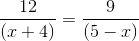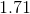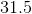The correct answer is. Cross multiplying the equation in the question will give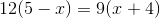. This is simplified to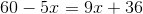. Combining like terms gives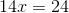. Finally, isolatinggives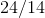or